Data Structures and Algorithms with Object-Oriented Design Patterns in C#Basics

A priority queue is a container which provides the following three operations:

Enqueue
used to put objects into the container;
Min
accesses the smallest object in the container; and
DequeueMin
removes the smallest object from the container.

A priority queue is used to store a finite set of keys drawn from a totally ordered set of keys K. As distinct from search trees, duplicate keys are allowed in priority queues.

Programdefines the PriorityQueue interface. The PriorityQueue interface extends the Container interface defined in Program. In addition to the inherited methods, the PriorityQueue interface comprises the three methods listed above.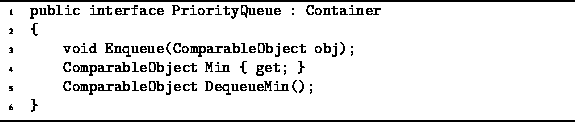Program: PriorityQueue interface.

Programdefines the MergeablePriorityQueue interface. The MergeablePriorityQueue interface extends the PriorityQueue interface defined in Program. A mergeable priority queue   is one which provides the ability to merge efficiently two priority queues into one. Of course it is always possible to merge two priority queues by dequeuing the elements of one queue and enqueueing them in the other. However, the mergeable priority queue implementations we will consider allow more efficient merging than this.Program: MergeablePriorityQueue interface.

It is possible to implement the required functionality using data structures that we have already considered. For example, a priority queue can be implemented simply as a list. If an unsorted list  is used, enqueueing can be accomplished in constant time. However, finding the minimum and removing the minimum each require O(n) time where n is the number of items in the queue. On the other hand, if a sorted list  is used, finding the minimum and removing it is easy--both operations can be done in constant time. However, enqueueing an item in a sorted list requires O(n) time.

Another possibility is to use a search tree. For example, if an AVL tree  is used to implement a priority queue, then all three operations can be done in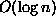time. However, search trees provide more functionality than we need. Search trees support finding the largest item with Max, deletion of arbitrary objects with Withdraw, and the ability to visit in order all the contained objects via DepthFirstTraversal. All these operations can be done as efficiently as the priority queue operations. Because search trees support more methods than we really need for priority queues, it is reasonable to suspect that there are more efficient ways to implement priority queues. And indeed there are!

A number of different priority queue implementations are described in this chapter. All the implementations have one thing in common--they are all based on a special kind of tree called a min heap or simply a heap.

Definition ((Min) Heap)  A (Min) Heap   is a tree,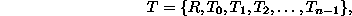with the following properties:

1. Every subtree of T is a heap; and,
2. The root of T is less than or equal to the root of every subtree of T. That is,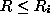for all i,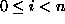, where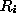is the root of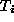.
According to Definition, the key in each node of a heap is less than or equal to the roots of all the subtrees of that node. Therefore, by induction, the key in each node is less than or equal to all the keys contained in the subtrees of that node. Note, however, that the definition says nothing about the relative ordering of the keys in the subtrees of a given node. For example, in a binary heap either the left or the right subtree of a given node may have the larger key.Copyright © 2001 by Bruno R. Preiss, P.Eng. All rights reserved.